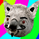Dilihat 44390
Pivot points simply took the high, low, and closing price from the previous period and
divided by 3 to find the pivot . From this pivot , traders would then base their
calculations for three support, and three resistance levels. The calculation for the most
basic flavor of pivot points , known as ‘floor-trader pivots’, along with their support and
resistance levels.
```////////////////////////////////////////////////////////////
//  Copyright by HPotter v1.0 10/07/2014
// Pivot points simply took the high, low, and closing price from the previous period and
// divided by 3 to find the pivot. From this pivot, traders would then base their
// calculations for three support, and three resistance levels. The calculation for the most
// basic flavor of pivot points, known as ‘floor-trader pivots’, along with their support and
// resistance levels.
////////////////////////////////////////////////////////////
study(title="Pivot Point", shorttitle="Pivot Point", overlay = true)
width = input(2, minval=1)
xHigh  = security(tickerid,"D", high)
xLow   = security(tickerid,"D", low)
xClose = security(tickerid,"D", close)
vPP = (xHigh+xLow+xClose) / 3
vR1 = vPP+(vPP-xLow)
vS1 = vPP-(xHigh - vPP)
vR2 = vPP + (xHigh - xLow)
vS2 = vPP - (xHigh - xLow)
vR3 = xHigh + 2 * (vPP - xLow)
vS3 = xLow - 2 * (xHigh - vPP)
plot(vS1, color=#ff0000, title="S1", style = circles, linewidth = width)
plot(vS2, color=#ff002a, title="S2", style = circles, linewidth = width)
plot(vS3, color=#ff014a, title="S3", style = circles, linewidth = width)
plot(vR1, color=#009600, title="R1", style = circles, linewidth = width)
plot(vR2, color=#006F00, title="R2", style = circles, linewidth = width)
plot(vR3, color=#004900, title="R3", style = circles, linewidth = width)
```

## KomentarHello,

I know this is a stupid question, but how can I add this to my charts? Haha

Thanks a lot!
Jawabandrewwhittle
@andrewwhittle, I guess you'll change TickerId from "D" to "W"?
Jawab